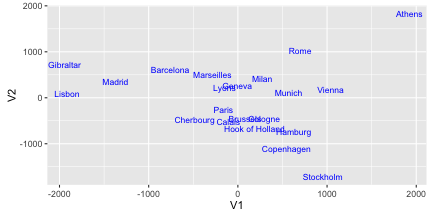This document explains PCA, clustering, LFDA and MDS related plotting using `{ggplot2}` and `{ggfortify}`.

# Plotting PCA (Principal Component Analysis)

`{ggfortify}` let `{ggplot2}` know how to interpret PCA objects. After loading `{ggfortify}`, you can use `ggplot2::autoplot` function for `stats::prcomp` and `stats::princomp` objects.

``````library(ggfortify)
df <- iris[c(1, 2, 3, 4)]
autoplot(prcomp(df))
``````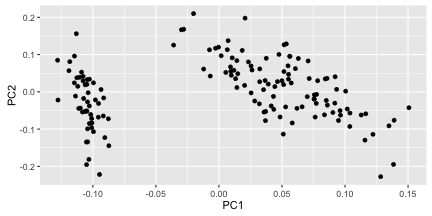PCA result should only contains numeric values. If you want to colorize by non-numeric values which original data has, pass original data using `data` keyword and then specify column name by `colour` keyword. Use `help(autoplot.prcomp)` (or `help(autoplot.*)` for any other objects) to check available options.

``````autoplot(prcomp(df), data = iris, colour = 'Species')
``````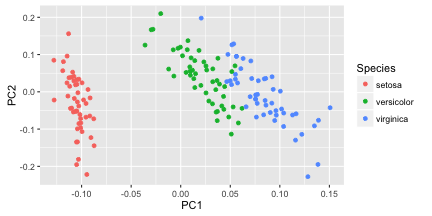Passing `label = TRUE` draws each data label using `rownames`

``````autoplot(prcomp(df), data = iris, colour = 'Species', label = TRUE, label.size = 3)
``````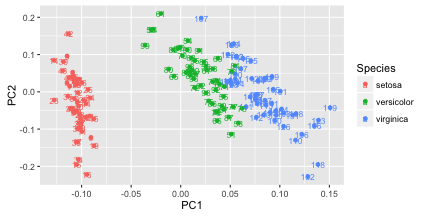Passing `shape = FALSE` makes plot without points. In this case, `label` is turned on unless otherwise specified.

``````autoplot(prcomp(df), data = iris, colour = 'Species', shape = FALSE, label.size = 3)
``````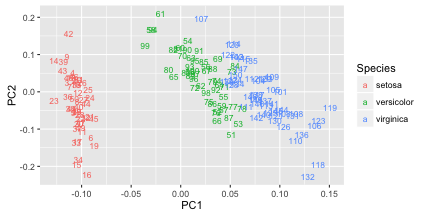Passing `loadings = TRUE` draws eigenvectors.

``````autoplot(prcomp(df), data = iris, colour = 'Species', loadings = TRUE)
``````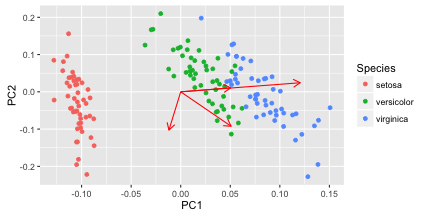You can attach eigenvector labels and change some options.

``````autoplot(prcomp(df), data = iris, colour = 'Species',
``````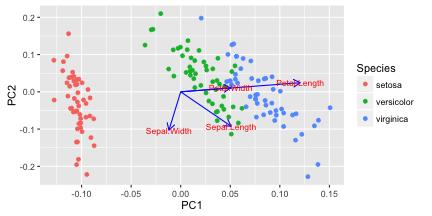By default, each component are scaled as the same as standard `biplot`. You can disable the scaling by specifying `scale = 0`

``````autoplot(prcomp(df), scale = 0)
``````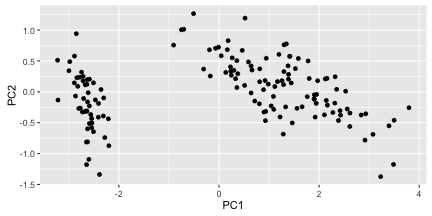# Plotting Factor Analysis

`{ggfortify}` supports `stats::factanal` object as the same manner as PCAs. Available opitons are the same as PCAs.

Important You must specify `scores` option when calling `factanal` to calcurate sores (default `scores = NULL`). Otherwise, plotting will fail.

``````d.factanal <- factanal(state.x77, factors = 3, scores = 'regression')
autoplot(d.factanal, data = state.x77, colour = 'Income')
``````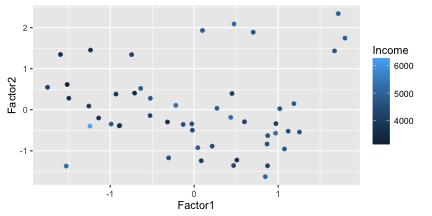``````autoplot(d.factanal, label = TRUE, label.size = 3,
``````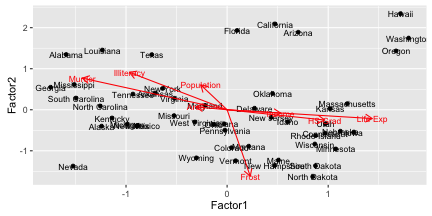# Plotting K-means

`{ggfortify}` supports `stats::kmeans` class. You must explicitly pass original data to `autoplot` function via `data` keyword. Because `kmeans` object doesn't store original data. The result will be automatically colorized by categorized cluster.

``````set.seed(1)
autoplot(kmeans(USArrests, 3), data = USArrests)
``````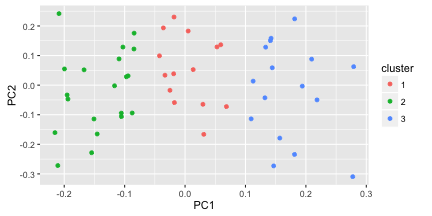``````autoplot(kmeans(USArrests, 3), data = USArrests, label = TRUE, label.size = 3)
``````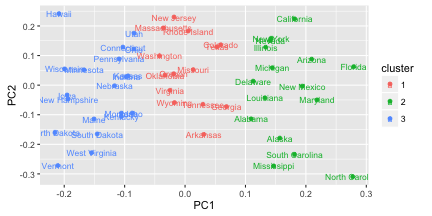# Plotting cluster package

`{ggfortify}` supports `cluster::clara`, `cluster::fanny`, `cluster::pam` classes. Because these instances should contains original data in its property, there is no need to pass original data explicitly.

``````library(cluster)
autoplot(clara(iris[-5], 3))
``````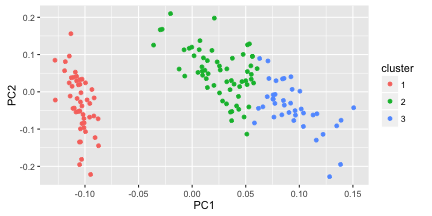Specifying `frame = TRUE` in `autoplot` for `stats::kmeans` and `cluster::*` draws convex for each cluster.

``````autoplot(fanny(iris[-5], 3), frame = TRUE)
``````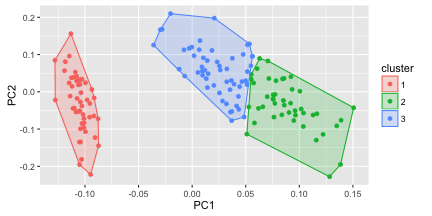If you want probability ellipse, `{ggplot2}` 1.0.0 or later is required. Specify whatever supported in `ggplot2::stat_ellipse`'s `type` keyword via `frame.type` option.

``````autoplot(pam(iris[-5], 3), frame = TRUE, frame.type = 'norm')
``````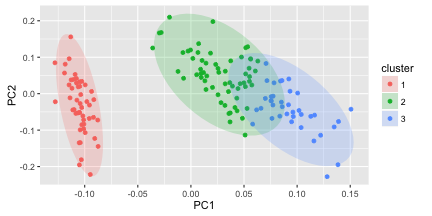# Plotting Local Fisher Discriminant Analysis with `{lfda}` package

`{lfda}` package supports a set of Local Fisher Discriminant Analysis methods. You can use `autoplot` to plot the analysis result as the same manner as PCA.

Thanks to the kind contribution of Yuan Tang, the author of `{lfda}` package.

``````library(lfda)

# Local Fisher Discriminant Analysis (LFDA)
model <- lfda(iris[-5], iris[, 5], r = 3, metric="plain")
autoplot(model, data = iris, frame = TRUE, frame.colour = 'Species')
``````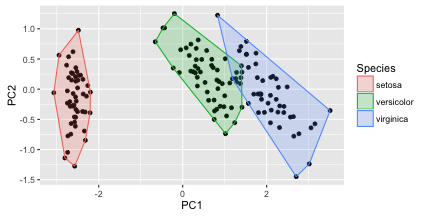``````# Semi-supervised Local Fisher Discriminant Analysis (SELF)
model <- self(iris[-5], iris[, 5], beta = 0.1, r = 3, metric="plain")
autoplot(model, data = iris, frame = TRUE, frame.colour = 'Species')
``````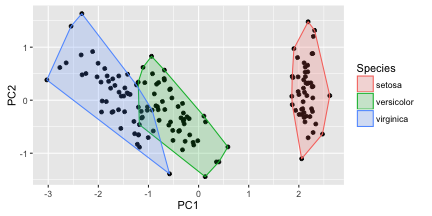# Plotting Multidimensional Scaling

## Before Plotting

Even though MDS functions returns `matrix` or `list` (not specific class), `{ggfortify}` can infer background class from `list` attribute and perform `autoplot`.

NOTE Inference from `matrix` is not supported.

NOTE `{ggfortify}` can plot `stats::dist` instance as heatmap.

``````autoplot(eurodist)
``````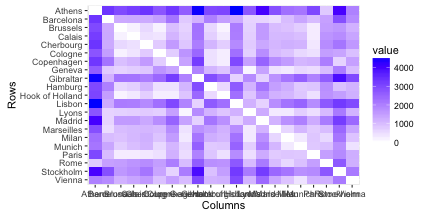## Plotting Classical (Metric) Multidimensional Scaling

`stats::cmdscale` performs Classical MDS and returns point coodinates as `matrix`, thus you can not use `autoplot` in this case. However, either `eig = TRUE`, `add = True` or `x.ret = True` is specified, `stats::cmdscale` return `list` instead of `matrix`. In these cases, `{ggfortify}` can infer how to plot it via `autoplot`. Refer to `help(cmdscale)` to check what these options are.

``````autoplot(cmdscale(eurodist, eig = TRUE))
``````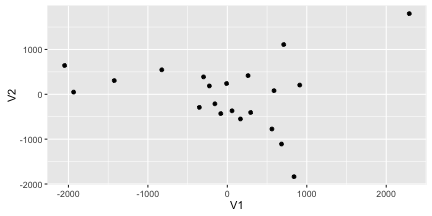Specify `label = TRUE` to plot labels.

``````autoplot(cmdscale(eurodist, eig = TRUE), label = TRUE, label.size = 3)
``````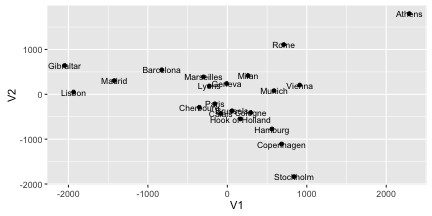## Plotting Non-metric Multidimensional Scaling

`MASS::isoMDS` and `MASS::sammon` perform Non-metric MDS and return `list` which contains point coordinates. Thus, `autoplot` can be used.

NOTE On background, `autoplot.matrix` is called to plot MDS. See `help(autoplot.matrix)` to check available options.

``````library(MASS)
autoplot(isoMDS(eurodist), colour = 'orange', size = 4, shape = 3)
``````
``````## initial  value 7.505733
## final  value 7.505688
## converged
``````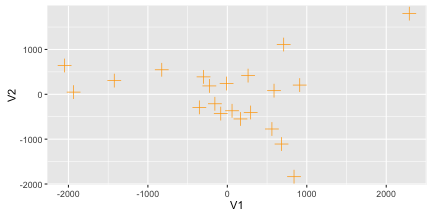Passing `shape = FALSE` makes plot without points. In this case, `label` is turned on unless otherwise specified.

``````autoplot(sammon(eurodist), shape = FALSE, label.colour = 'blue', label.size = 3)
``````
``````## Initial stress        : 0.01705
## stress after  10 iters: 0.00951, magic = 0.500
## stress after  20 iters: 0.00941, magic = 0.500
``````